## General Chemistry

When discussing the Bohr’s model of the hydrogen atom, we saw that absorbing light with sufficient energy moves the electron to a higher energy level, and when it falls back to a lower energy level, light is emitted as energy is lost.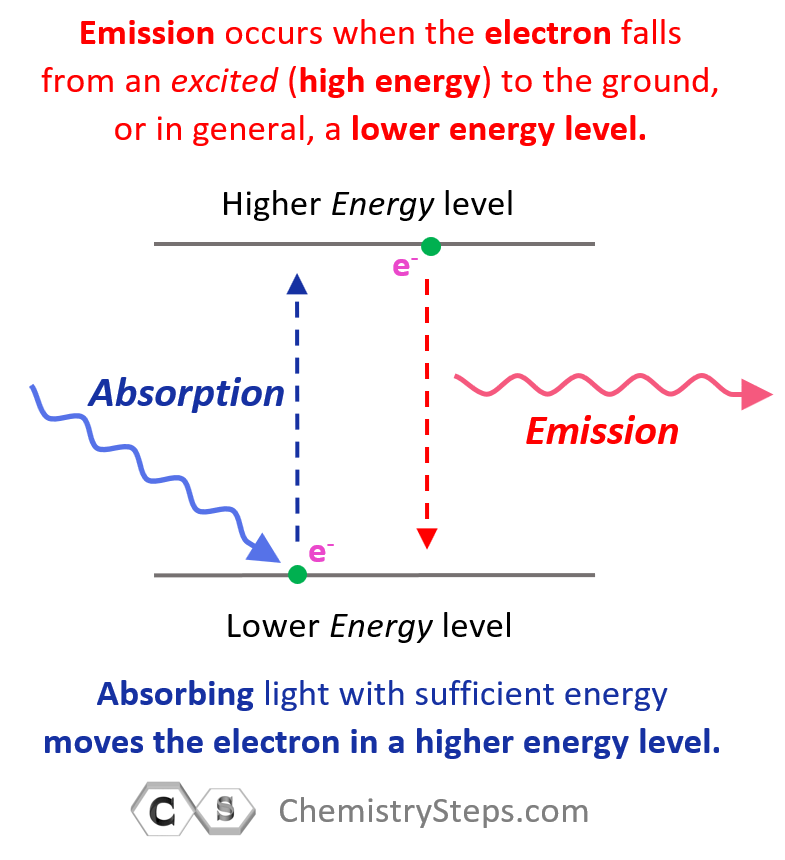Next, in the photoelectric effect, we saw that if the photon striking an electron has higher energy than the energy level of the greatest orbit, the electron is ejected from the atom as it passes through all the energy barriers associated it the orbital energy levels: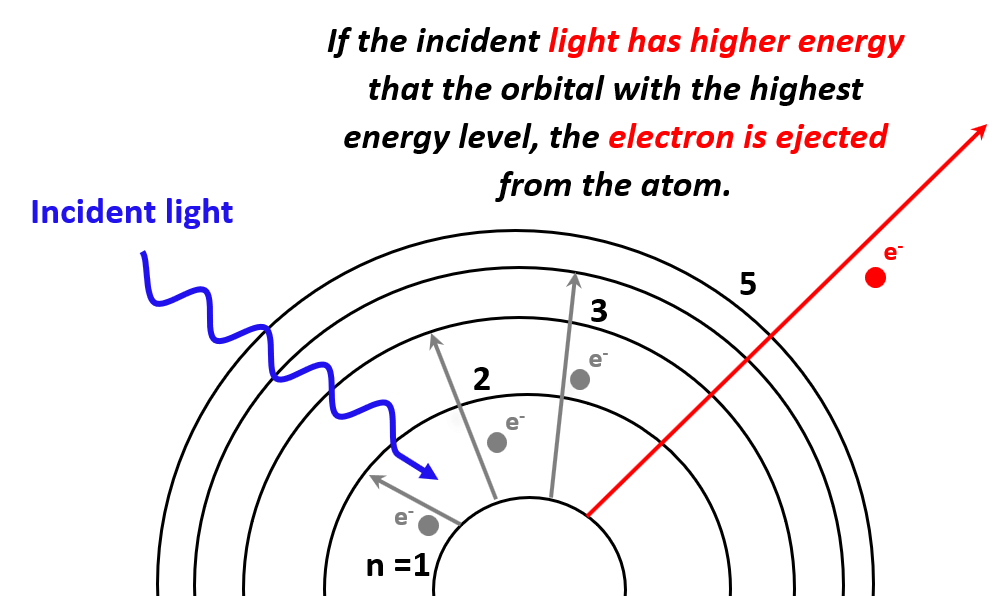The minimum energy required to expel an electron from the element (generally a metal) is called the work function (Φ) and is characteristic of each metal.

The concept of ionization energy that we will discuss in this article is similar to this phenomenon, so let’s see what it is and how it is different from the photoelectric effect.

# The Ionization Energy

Applying the same principle as in the photoelectric effect, electrons can also be removed from isolated atoms in a gaseous state. The amount of energy required to remove an electron from the isolated neutral atom in the gaseous state is called the atom’s ionization energy (Ei or IE).In this image, the hydrogen atom was ionized by ejecting its one electron.

For multielectron atoms, there are different ionization energies depending on which electron is removed. The energy necessary to remove the first electron in the outermost valence shell is the first ionization energy (Ei1), for the second electron, we have Ei2, and so forth.

Let’s compare the ionization energies of aluminum: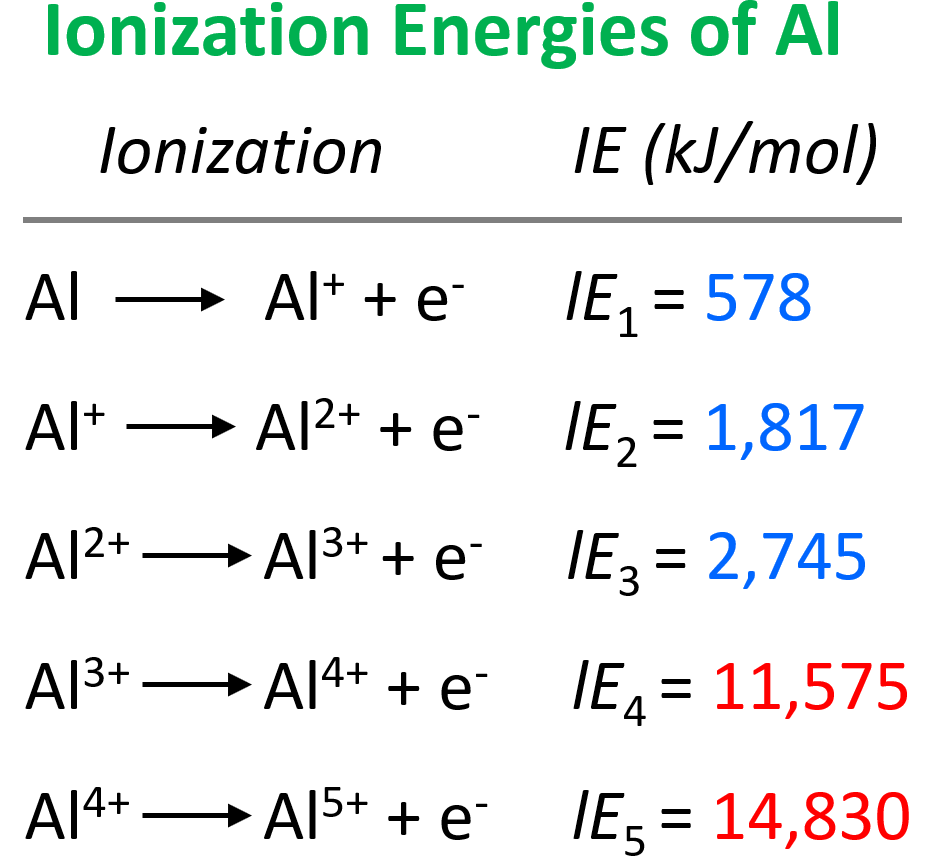The main observation from the values of ionization energies is that they get larger as we move on from the first electron. The first electron is removed from a neutral atom, while all the subsequent ones are removed from an ion. Therefore, we can correlate the values of ionization energies with the charge of the ion. As the charge increases, the ionization energy increases as well:It is more difficult to remove an electron from the Al1+ ion than from the Al2+ ion, and we can explain this by the increasing electrostatic forces between the higher-charged cation and the electron.

Let’s now make a graph to see if there is any other pattern when comparing the changes in ionization energies: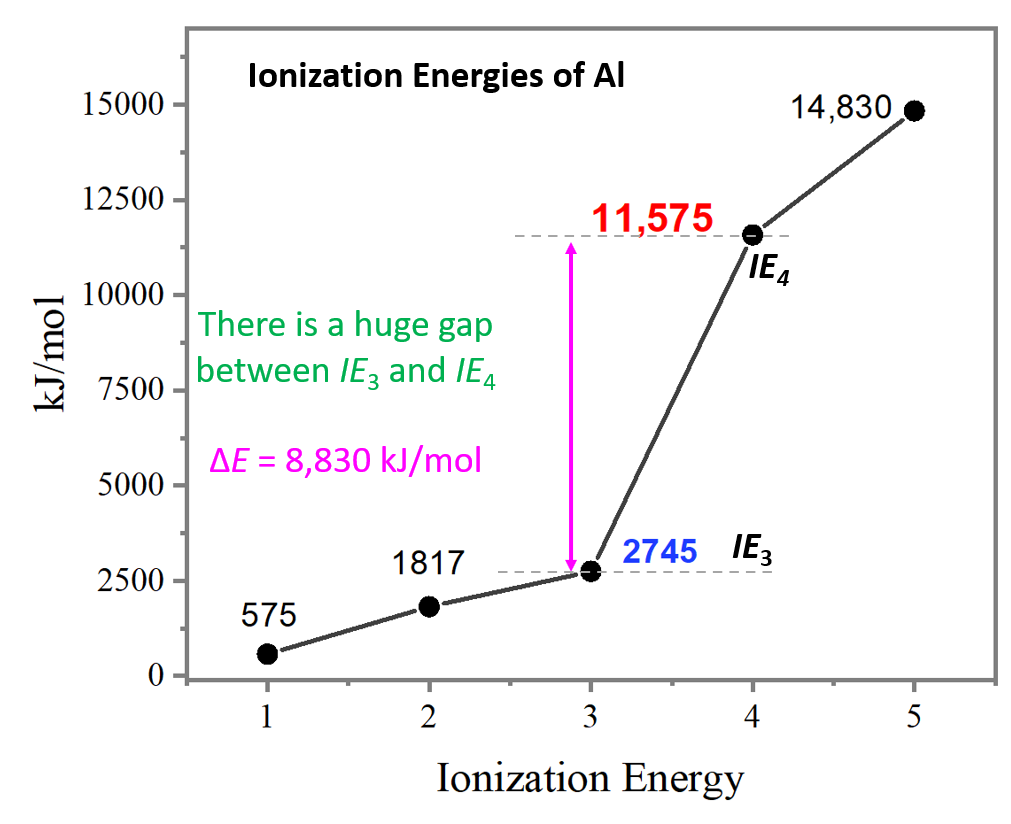Notice the huge jump going from the third to the fourth ionization energies. It is a lot larger than the difference between IE1 and IE2 or IE2 and IE3.

So, why is there such a big difference between the third to fourth ionization energies of aluminum?

To answer this question, we need to look at the electron configuration of the element: Al – 1s22s22p63s23p1: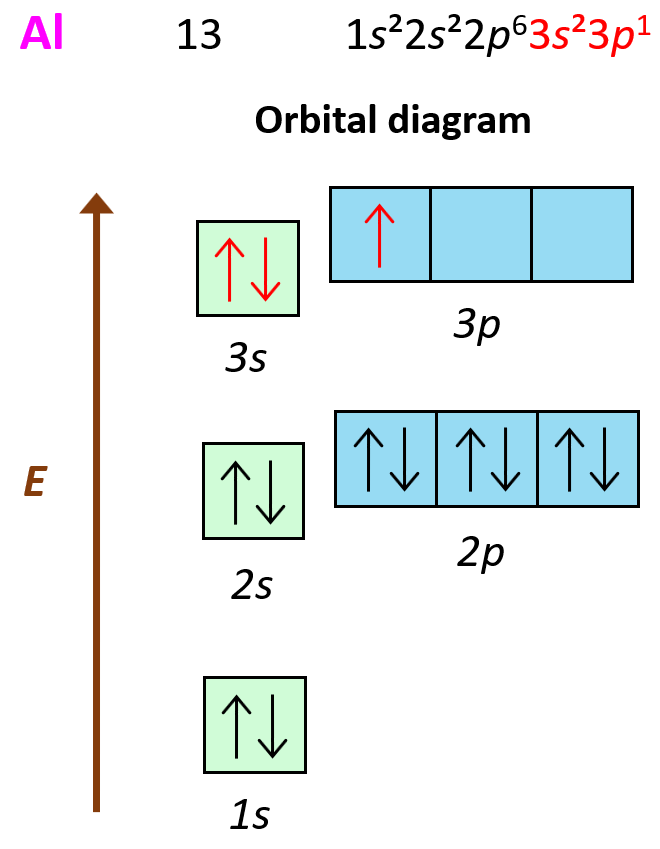What is important here is that the first three electrons that are removed are the valence electrons of Al as they are in the outermost main energy level (n = 3). Remember, these are the farthest away from the nuclei and therefore, the easiest to remove. Another factor affecting the ionization energy is the shielding by the electrons. In addition to the core electrons, the 3p1 electrons is also shielded by the 3s electrons while the latter is only shielded by the core electrons. So, even though removing the 3p1 electron will still require less energy than the 3s2 and 3s1 electrons, because the p sublevel is higher in energy (farther from the nuclei), this difference is a lot smaller than when we start removing the inner electrons of the atom.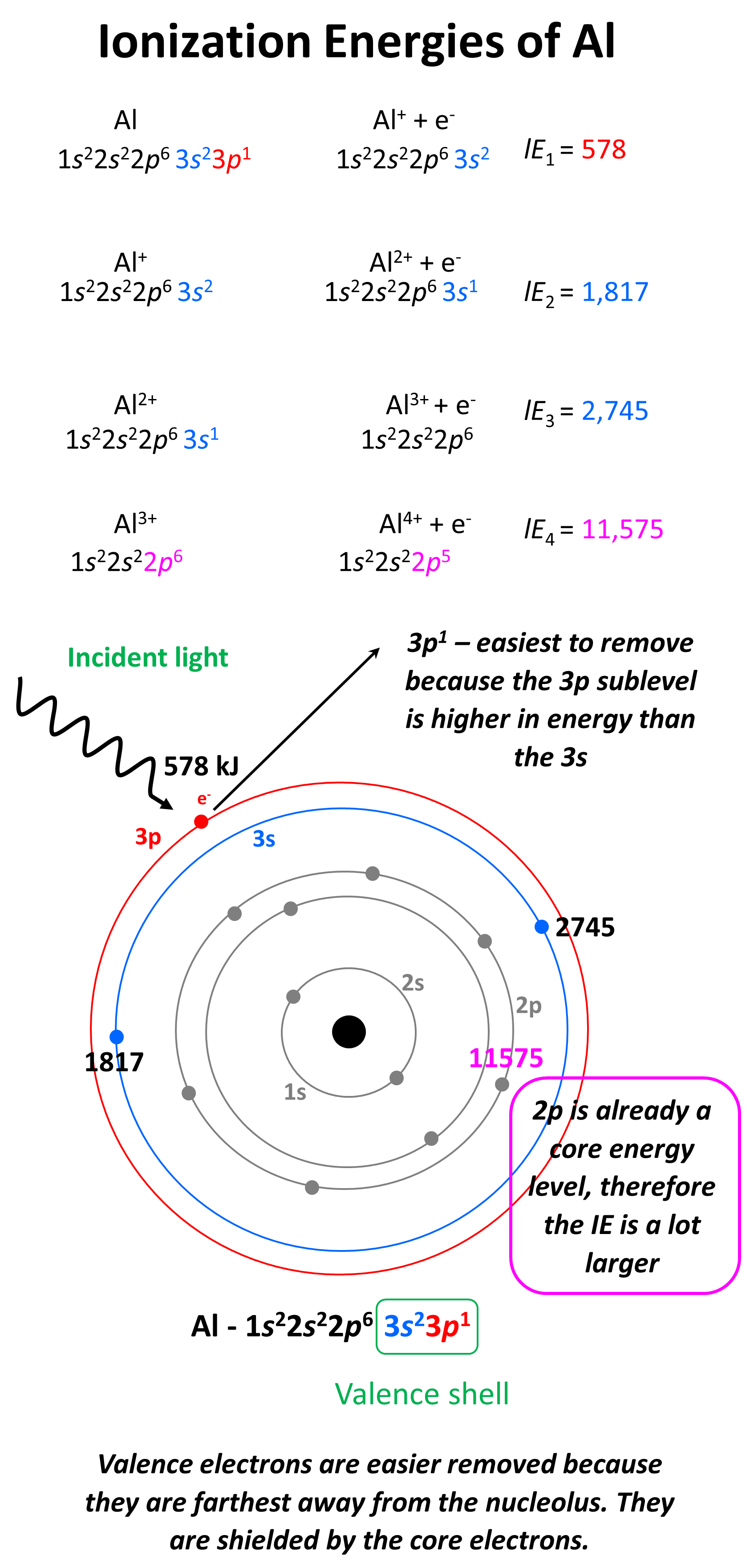Removing the second 3s electron is still more difficult because the charge of the ion gets larger, however, the difference is not as much as switching from 3s to 2s main energy level. So, to summarize this part, we conclude that it is a lot more difficult to remove core (inner) electrons than valence electrons, because of the ionic charge, distance, and shielding of the electron. This is expected as we know that it is the valence electrons that participate in chemical reactions because they are the easiest to remove and therefore, participate in bonding with another element.

# Periodic Trends of Ionization Energy

The first thing is to remember that ionization energy increases going up the periodic table because the atoms get smaller, and the electrons are closer to the nucleus. Also, smaller atoms have fewer electrons which decreases the shielding of the electron being removed. Let’s see this by comparing the first ionization energy of Li, K, and Na: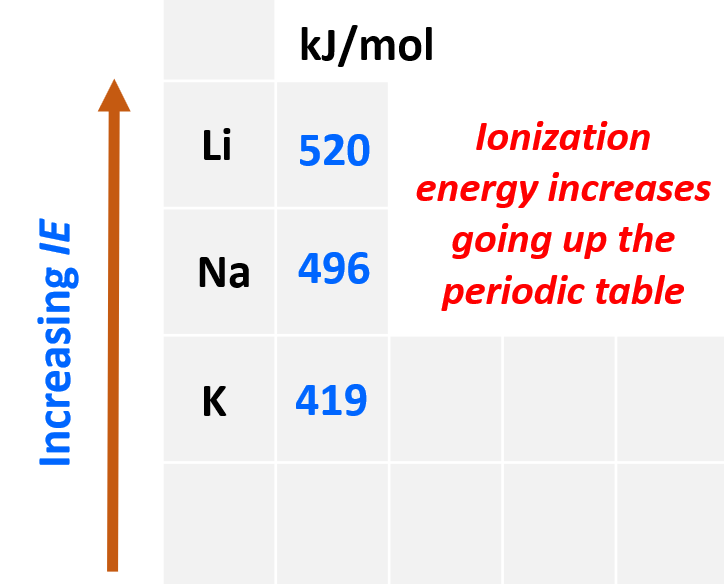Even though Na has a higher nuclear charge than Li (+11 vs +3), it takes less energy to remove its first electron. This is counterintuitive if we only compare the nuclear charges, however, the distance and the shielding of the electron turned out to be a greater factor, and because Na is a larger atom, its ionization energy is smaller.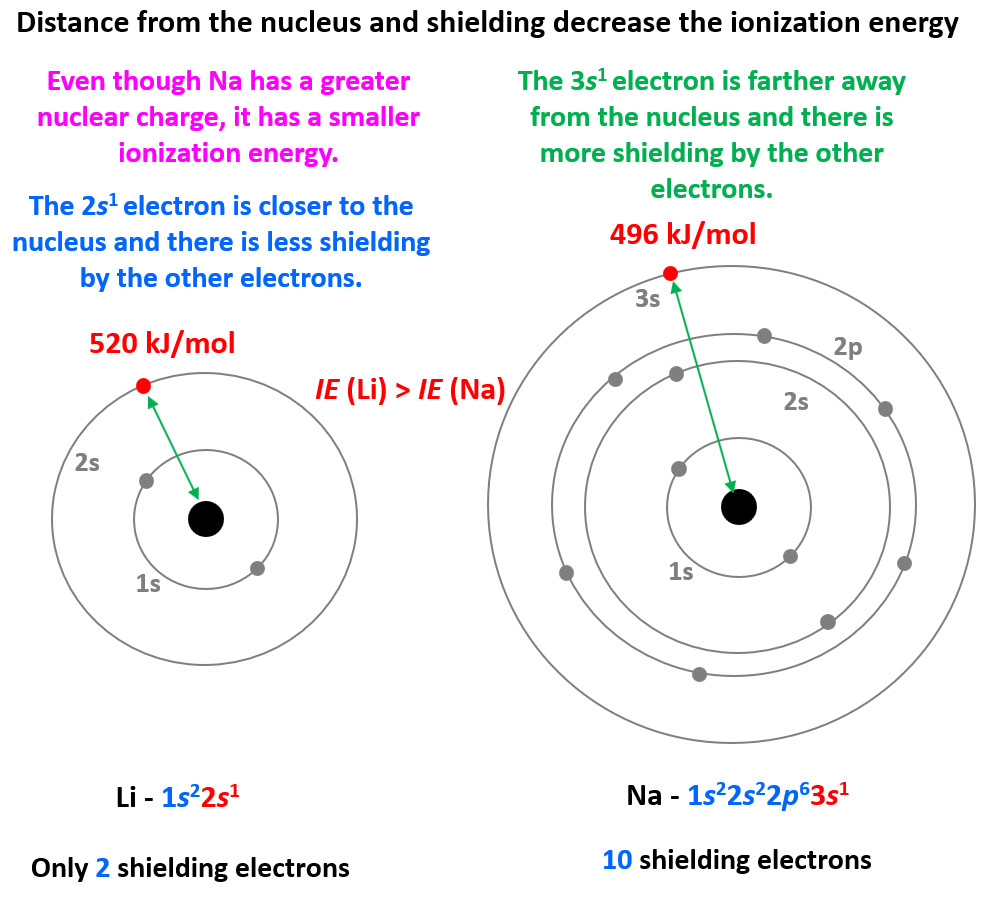So, in general, the more energy levels the atom has, the smaller its ionization energy gets because they add shielding from the nucleus.

The second pattern is the general increase of the ionization energy going to the right in the periodic table.

To explain this, let’s consider the elements in the 3rd row of the periodic table: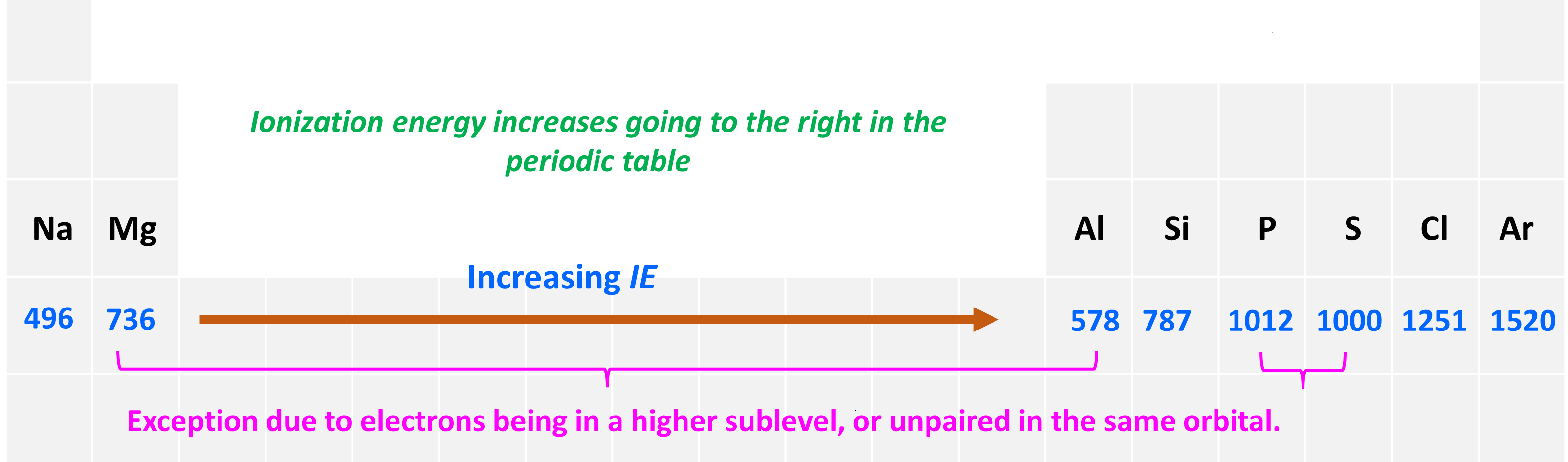First, let’s realize that all the elements are in the same row of the periodic table and therefore the valence electrons are in the same main energy level (n = 3). What is different though is their nuclear charge. The atomic number, and thus the number of protons, increases as we go to the right.

The big picture here is that going from left to right increases the ionization energy, although this is not true for comparing any two elements. For example, contrary to what one may expect based on the general trend, Al has smaller ionization energy than Mg, and so does sulfur compared to phosphorous. So, let’s understand why Mg has a higher ionization energy than Al.

The truth is we have already addressed this above when discussing the energy sublevels, so you can take a few minutes to try answering this question.

The reason is that the last valence electron of Al is 3p1 (1s22s22p63s23p1) while that of Mg is 3s2 (1s22s22p63s2). Remember, the p sublevel of a given energy level is farther away from the nucleus and therefore it is easier to remove: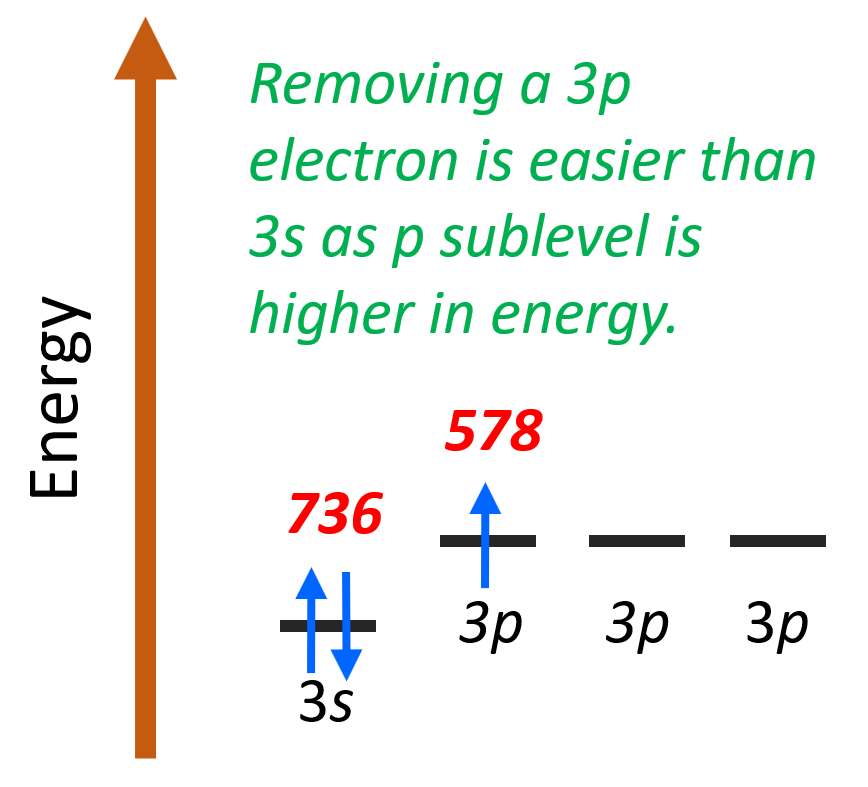This is a general pattern of p electrons having lower ionization energy than s electrons in the same energy level.

Next is the discrepancy of ionization energies of sulfur compared to phosphorous. S is more to the right and is expected to have a larger ionization energy, but it does not. Let’s look at the electron configurations of these elements – P: (1s22s22p63s23p3), S – (1s22s22p63s23p4).

It takes a little less energy to remove the 3p4 electron of sulfur (1000 kJ/mol) than the 3p3 electron of phosphorus (1012 kJ/mol). The reason for this is the repulsion forces between two electrons in the same orbital. Remember, the Hund’s rule, which states that electrons will fill all the degenerate orbitals (equal in energy) with parallel spins (both arrows up or down) first before pairing up in one orbital. So, in other words, if possible, electrons do not like to be in the same orbital.

So, going back to the ionization energy, if the 3p4 electron does not want to be (higher energy) in the same orbital with the 3p3 electron, then it will be easier to remove it than the 3p3 electron which is by itself in the orbital.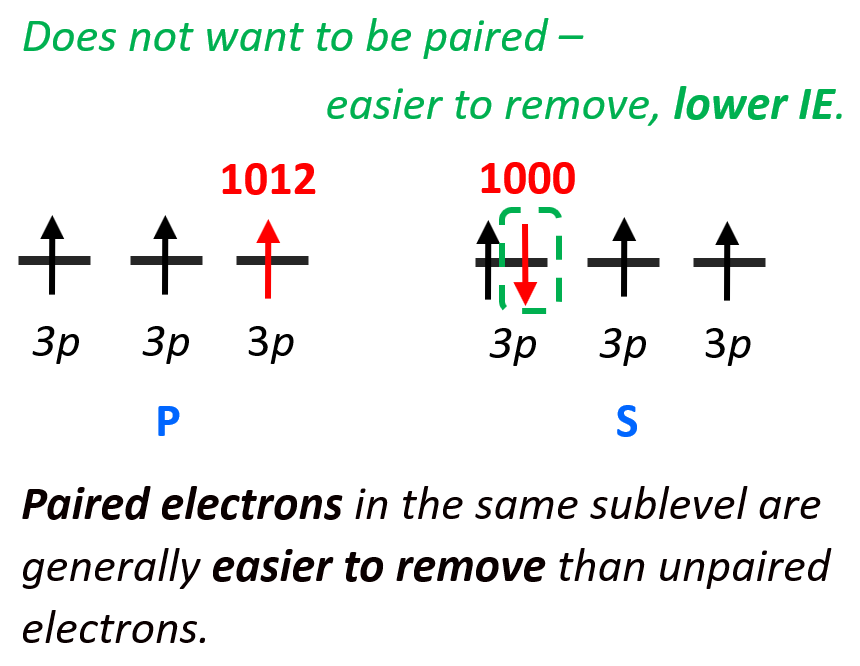Remember, lower energy means higher stability, and vice versa, if the electron (or anything else) has higher energy it is less stable, and therefore, easier to remove. So, paired electrons in the same sublevel are generally easier to remove than unpaired electrons.

Summarizing the patterns of ionization energies in the periodical table, remember that they tend to increase up and to the right of the periodic table: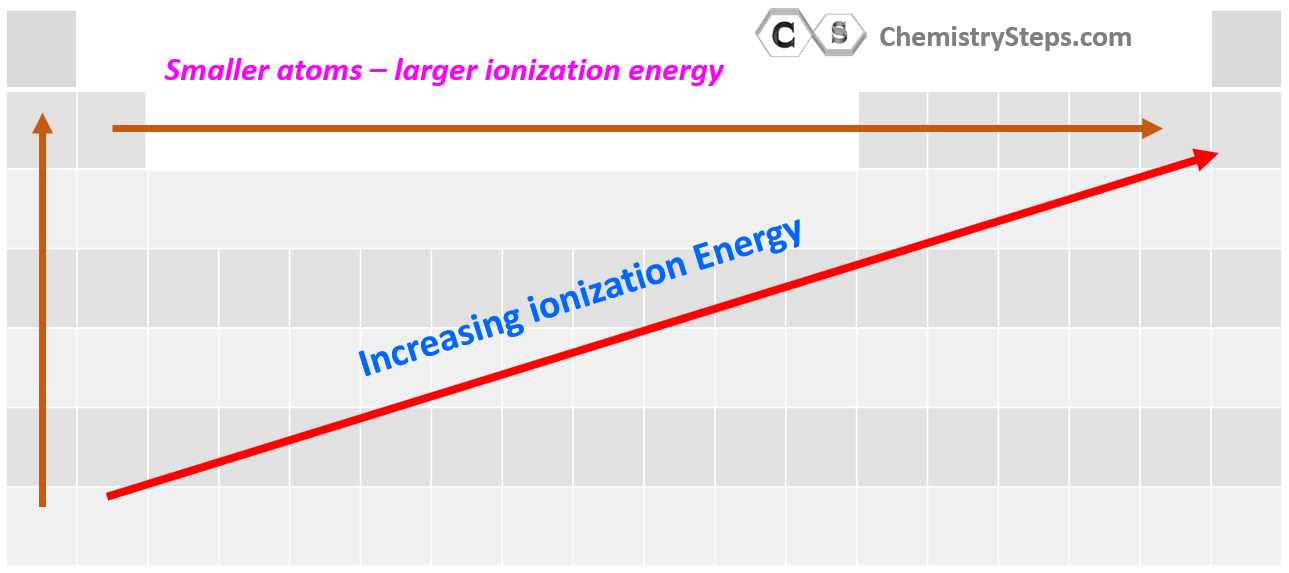# Examples:

1) Which atom in the following pairs has higher ionization energy: a) S or Cl, b) Si or Ge, c) Mg or Ca, d) N or O, e) Ga or Si

a) Both elements are in the same row and because ionization energies increase to the right of the periodic table, Cl has a larger ionization energy than sulfur.

b) Ionization energies increase moving up in the periodic table, therefore, silicon will have a larger ionization energy than germanium.

c) Ionization energies increase moving up in the periodic table, therefore, magnesium will have a larger ionization energy than calcium.

d) Although oxygen is more to the right in the same row and is expected to have a larger ionization energy, this pair falls into one of the exceptions because of the repulsion between electrons when they occupy the same orbital. This is the same principle as we discussed when comparing the ionization energies of phosphorus and sulfur.

The 3p4 electron of oxygen does not want to be (higher energy) in the same orbital with the 3p3 electron, so it will be easier to remove it than the 3p3 electron which is by itself in the orbital.2) Would you expect lithium or beryllium to have a larger second ionization energy?

In general, you can predict the subsequent ionization energies based on the number of valence electrons. Remember, these are the easiest to remove, and therefore, the element with more valence electrons will have relatively lower ionization energies until all the valence electrons are removed.

Beryllium is in group 2a, and therefore, has two valence electrons, while lithium has only one. Therefore, the beryllium will have a lower second ionization energy than Li (1757 kJ/mol vs 7298 kJ/mol).

We can also see this from the electron configurations:

Li: 1s22s1

Be: 1s22s2

The two electrons of Be are in the 2s orbital, while the second electron of Li needs to be removed from the 1s orbital which will require more energy.

Check Also